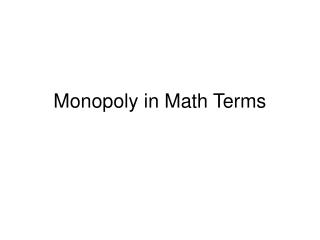DownloadDownload PresentationMonopoly in Math Terms

# Monopoly in Math Terms

Download Presentation## Monopoly in Math Terms

- - - - - - - - - - - - - - - - - - - - - - - - - - - E N D - - - - - - - - - - - - - - - - - - - - - - - - - - -
##### Presentation Transcript

1. Monopoly in Math Terms

2. Say for a monopoly Demand is P = 100 – 2Q We have a general rule when demand is in inverse form P = A – BQ, the MR = A – 2BQ. So for this specific monopoly MR = 100 – 4Q. TC = 10 + 2Q Set MR = MC and solve for Q to get the profit maximizing level of output. MR = 100 – 4Q = 2 = MC (remember cost from the section in Competition) Q = 98/4 = 24.5, Set this Q into demand to get the price, P = 100 – 2[24.5] = 51. Profit = TR-TC = 51(24.5) – 10 – 2[24.5] = 1249.5 – 59 = 1190.5